# 2 Circle Venn Diagram. Venn Diagram Example

## Venn's construction for 2 sets

This sample was created in ConceptDraw DIAGRAM diagramming and vector drawing software using the Venn Diagrams Solution from the "Diagrams" area of ConceptDraw Solution Park.

This example shows the 2 Circle Venn Diagram. The Venn Diagrams visualize all possible logical intersections between several sets. On this example you can see the intersections of 2 sets A and B. Venn Diagrams are widely used in mathematics, logic, statistics, marketing, sociology, etc.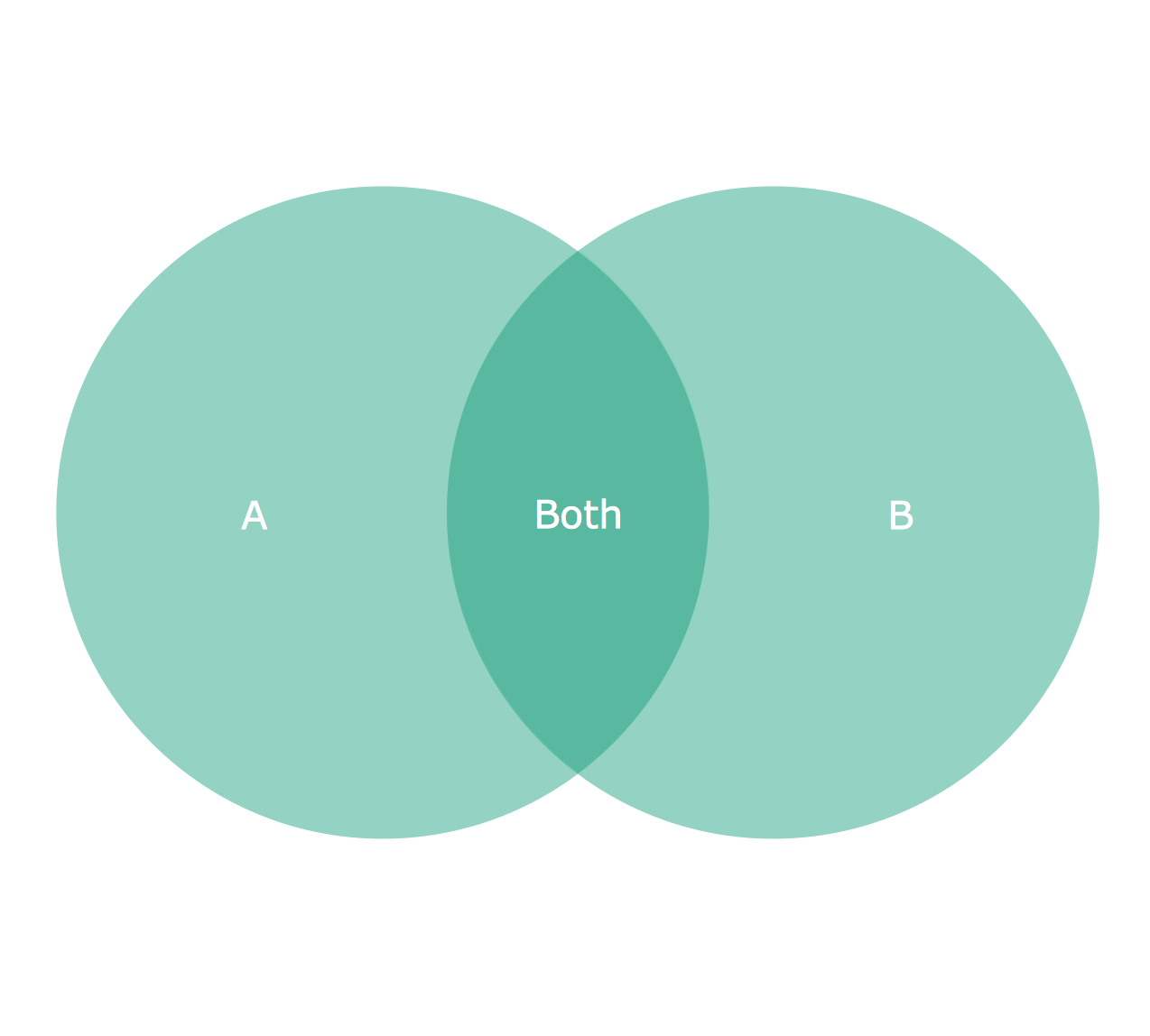Example 1. 2 Circle Venn Diagram.

Using the ready-to-use predesigned objects, samples and templates from the Venn Diagrams Solution for ConceptDraw DIAGRAM you can create your own professional looking Venn Diagrams quick and easy.

The Venn Diagrams produced with ConceptDraw DIAGRAM are vector graphic documents and are available for reviewing, modifying, and converting to a variety of formats (image, HTML, PDF file, MS PowerPoint Presentation, Adobe Flash or MS Visio).

Related Solution:

### 3 Circle Venn Diagram. Venn Diagram Example

This template shows the Venn Diagram. It was created in ConceptDraw DIAGRAM v12 diagramming and vector drawing software using the ready-to-use objects from the Venn Diagrams Solution from the "What is a Diagram" area of ConceptDraw Solution Park. Venn Diagrams visualize all possible logical intersections between several sets and are widely used in mathematics, logic, statistics, marketing, sociology, etc.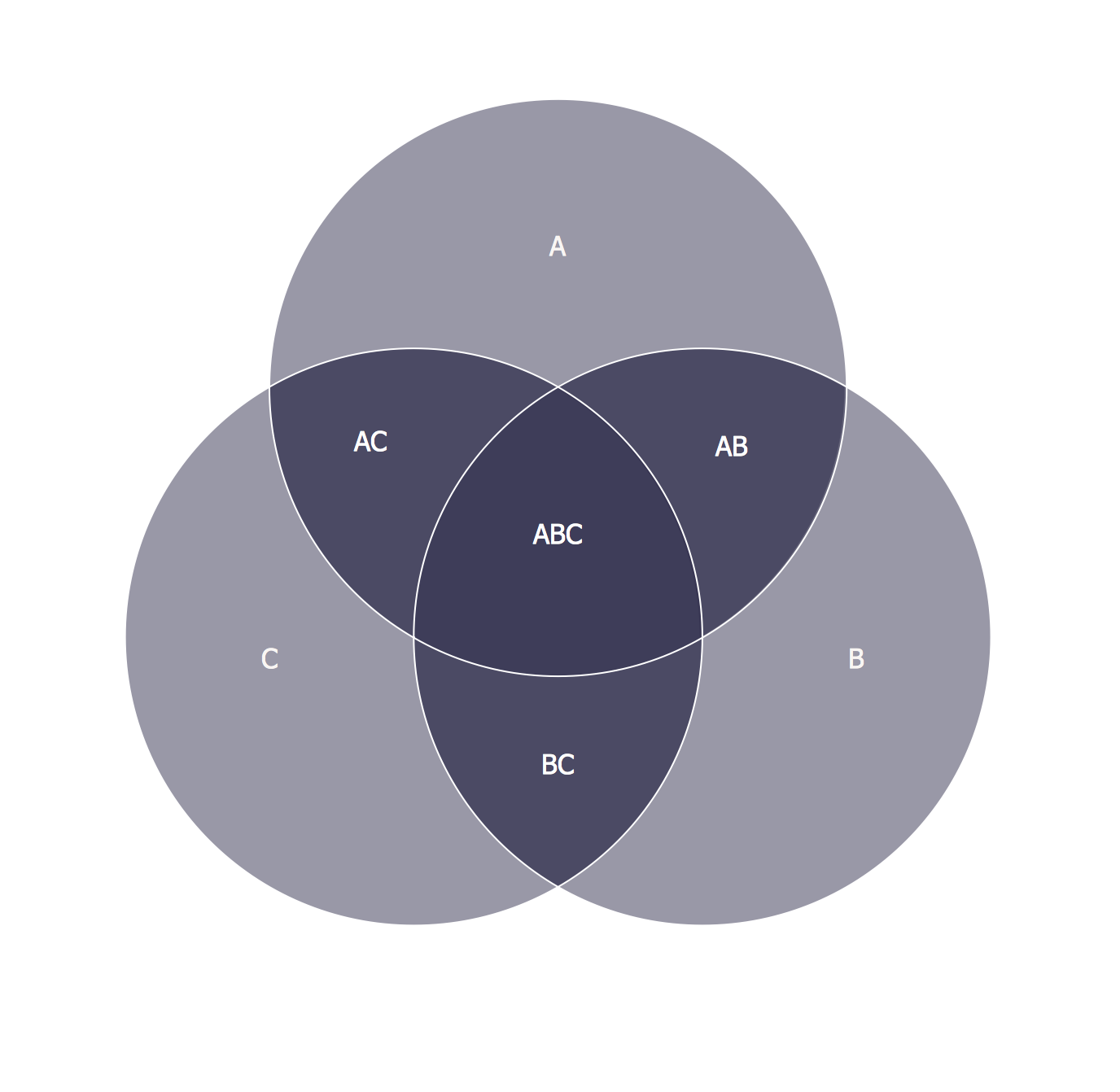Picture: 3 Circle Venn Diagram. Venn Diagram Example
Related Solution:

### Circle Diagrams

The Circle Diagrams are the diagrams which represent the central element surrounded by other items in a circle. This type of diagram is very popular and widely used in marketing and management, for process modeling, market, resource, time and cost analysis, for visualization causes and effects. If you dream to design the Circle Diagrams quick and easy â€“ the ConceptDraw DIAGRAM v12 software extended with Circle-Spoke Diagrams Solution from the "What is a Diagram" Area is exactly what you need.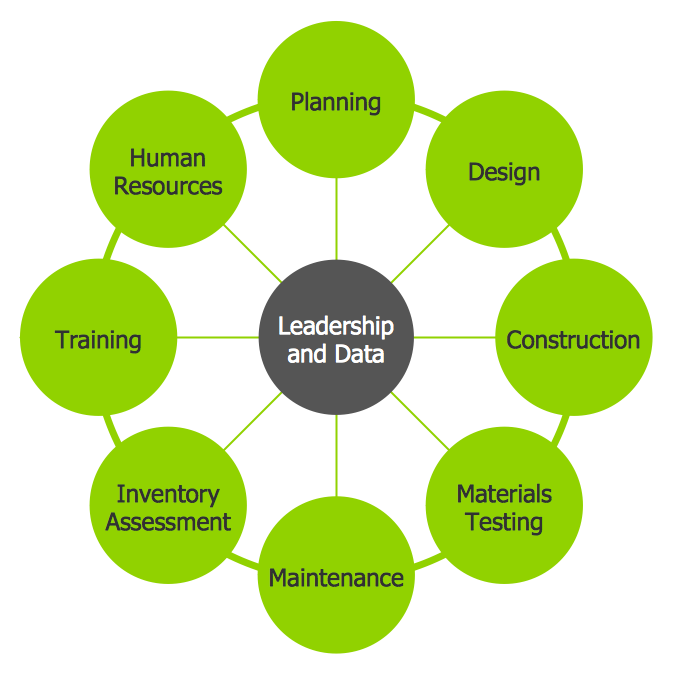Picture: Circle Diagrams
Related Solution:

### Venn Diagram Examples for Problem Solving.Venn Diagram as a Truth Table

Venn diagrams are illustrations used in the branch of mathematics known as set theory. They show the mathematical or logical relationship between different groups of things (sets). A Venn diagram shows all the possible logical relations between the sets.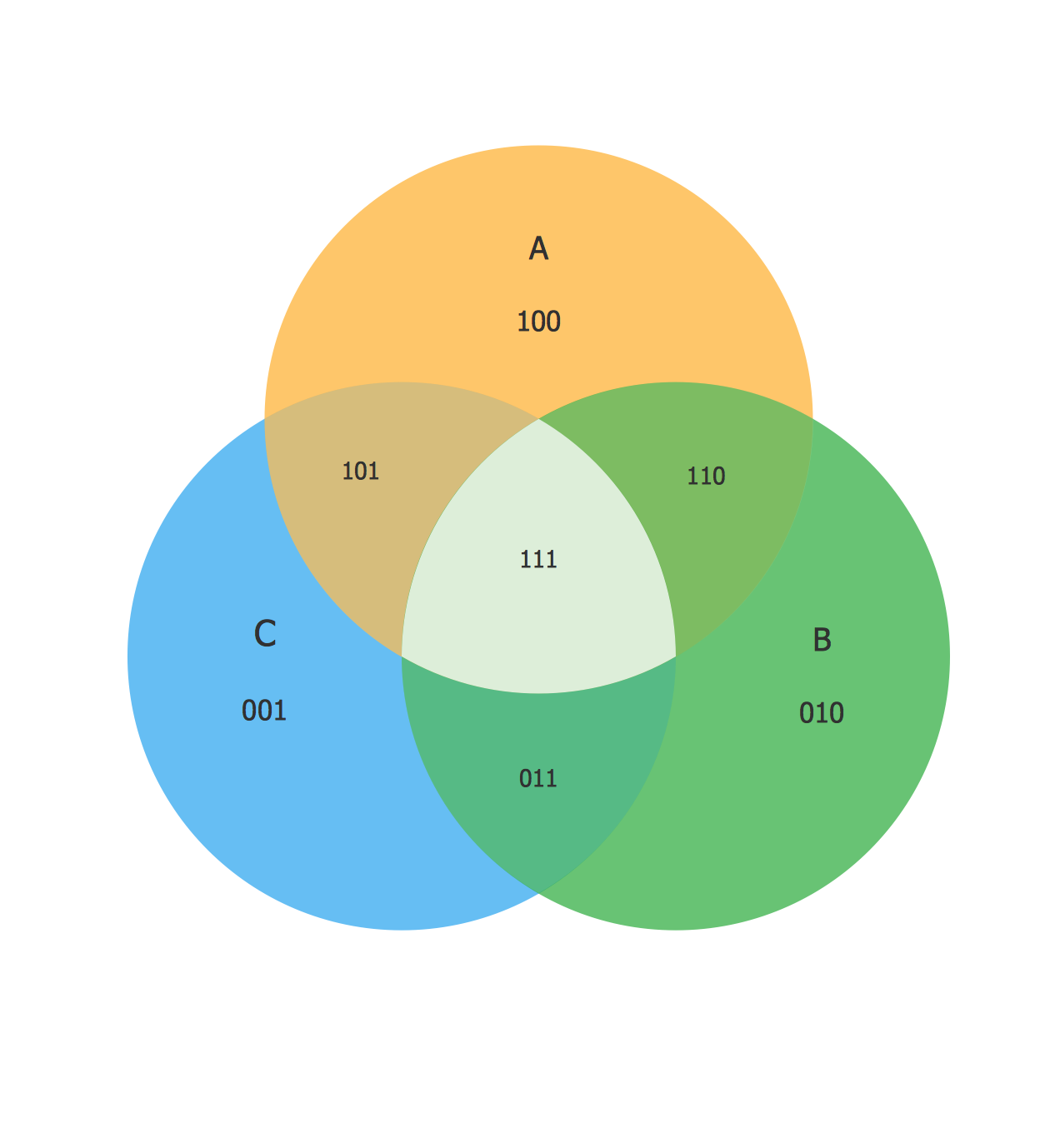Picture: Venn Diagram Examples for Problem Solving.Venn Diagram as a Truth Table
Related Solution:

### Cylinder Venn Diagram

You need design Cylinder Venn Diagram? Nothing could be easier with ConceptDraw DIAGRAM v12 diagramming and vector drawing software extended with Venn Diagrams Solution from the â€śWhat is a Diagramâ€ť Area. ConceptDraw DIAGRAM v12 allows you to design various Venn Diagrams including Cylinder Venn Diagrams.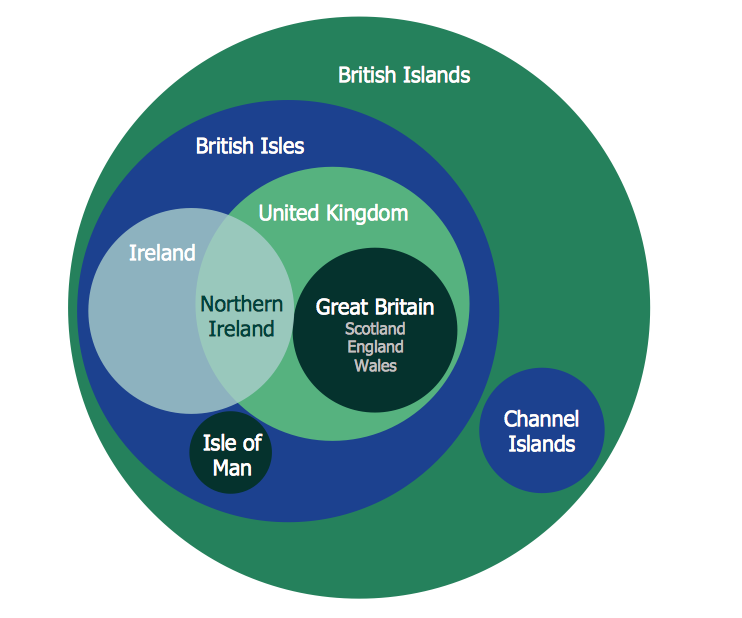Picture: Cylinder Venn Diagram
Related Solution:

### 3 Circle Venn. Venn Diagram Example

This example shows the 3 Circle Venn Diagram. The Venn Diagrams visualize all possible logical intersections between several sets. On this example you can see the intersections of 3 sets. Venn Diagrams are widely used in mathematics, logic, statistics, marketing, sociology, etc.Picture: 3 Circle Venn. Venn Diagram Example
Related Solution:

### Venn Diagram

Venn diagrams are illustrations used in the branch of mathematics known as set theory. They show the mathematical or logical relationship between different groups of things (sets). A Venn diagram shows all the possible logical relations between the sets.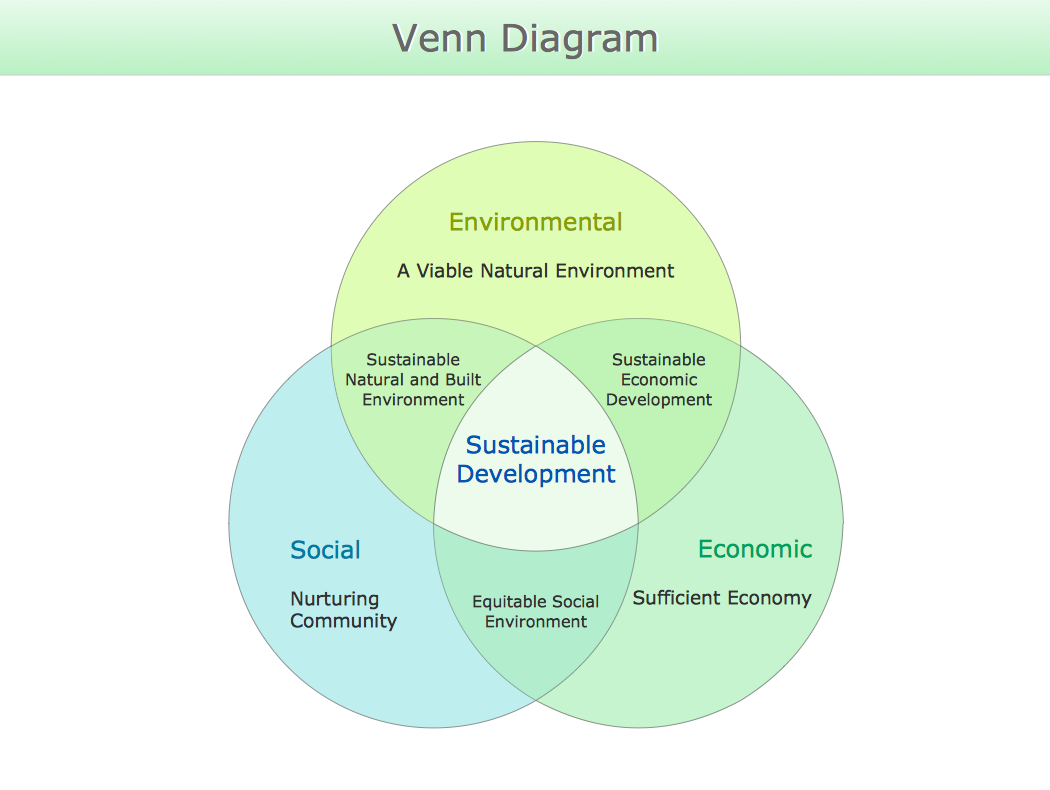Picture: Venn Diagram
Related Solution:

### Basic Circles Venn Diagram. Venn Diagram Example

Venn Diagrams visualize all possible logical relations between several sets and are widely used in mathematics, logic, statistics, marketing, sociology, etc. This Venn Diagram shows the relative complement of the set A with respect to the set B. It is the set of the elements in B, but not in A. In other words it is the set-theoretic difference B-A.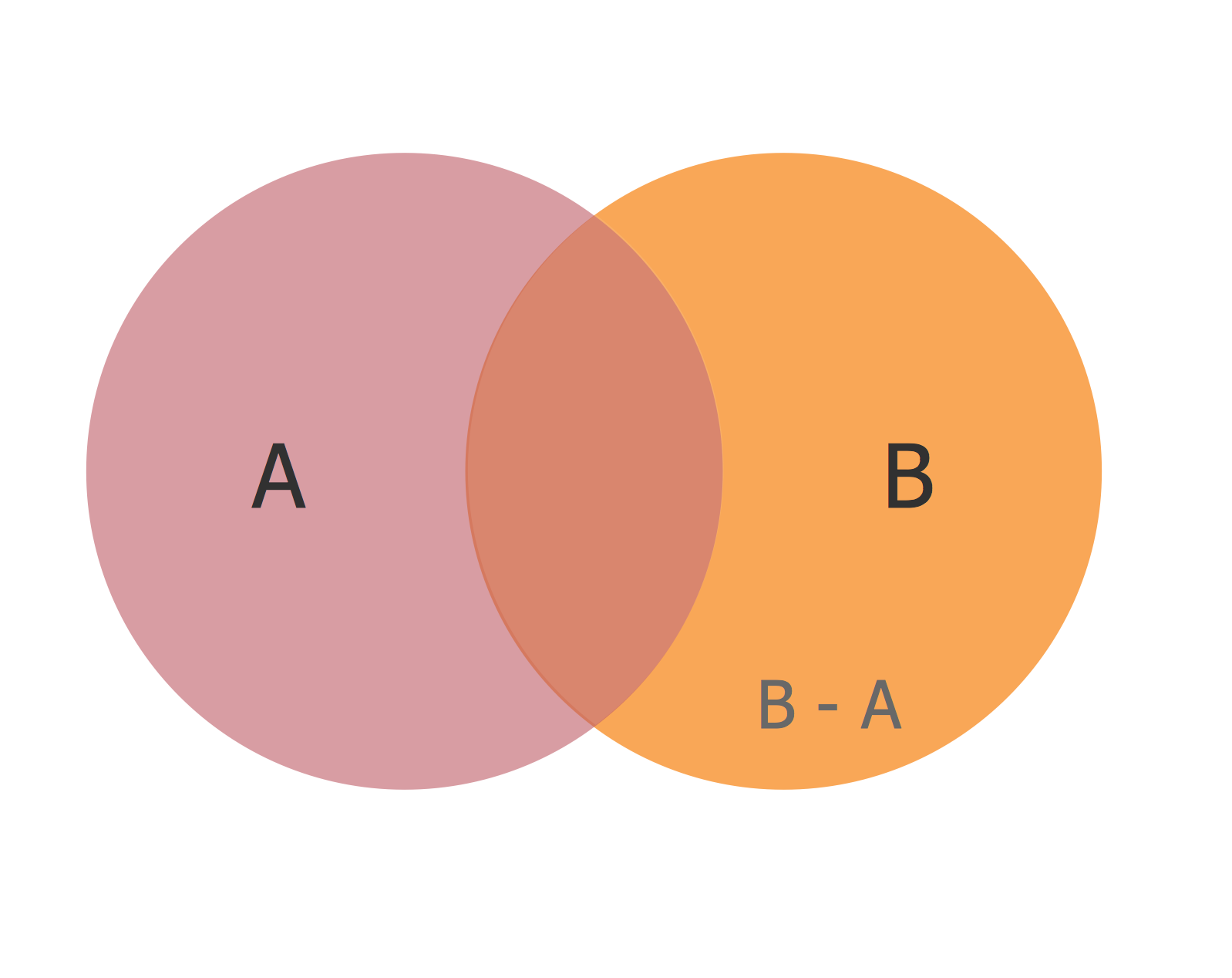Picture: Basic Circles Venn Diagram. Venn Diagram Example
Related Solution:

### Circle Spoke Diagram Template

ConceptDraw DIAGRAM v12 diagramming and vector drawing software offers the Circle Spoke Diagram Template included in the Circle-Spoke Diagrams Solution from the "What is a Diagram" Area. Use of ready template is the easiest way of drawing professional looking Circle-Spoke Diagrams.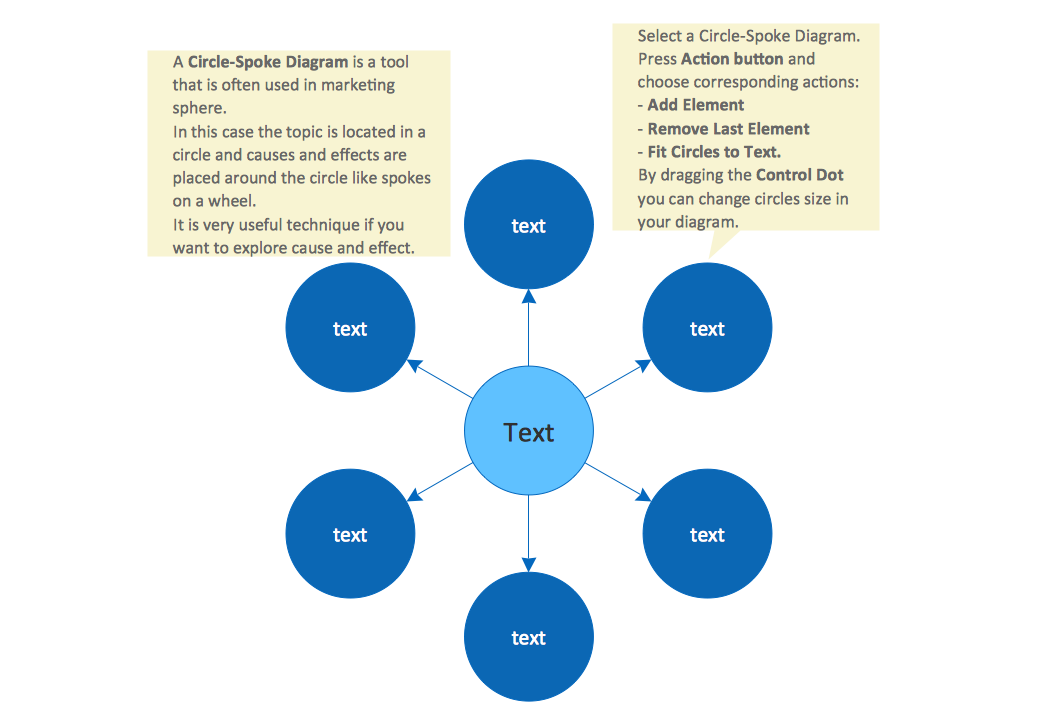Picture: Circle Spoke Diagram Template
Related Solution:

### Circular Diagram

Circular Diagram is a type of diagram widely used in marketing and economics for visualization information in a clear and visual form. ConceptDraw DIAGRAM v12 diagramming and vector drawing software offers the useful tools of the Target and Circular Diagrams solution from the Marketing area of ConceptDraw Solution Park for effective drawing a Circular Diagram of any complexity and design.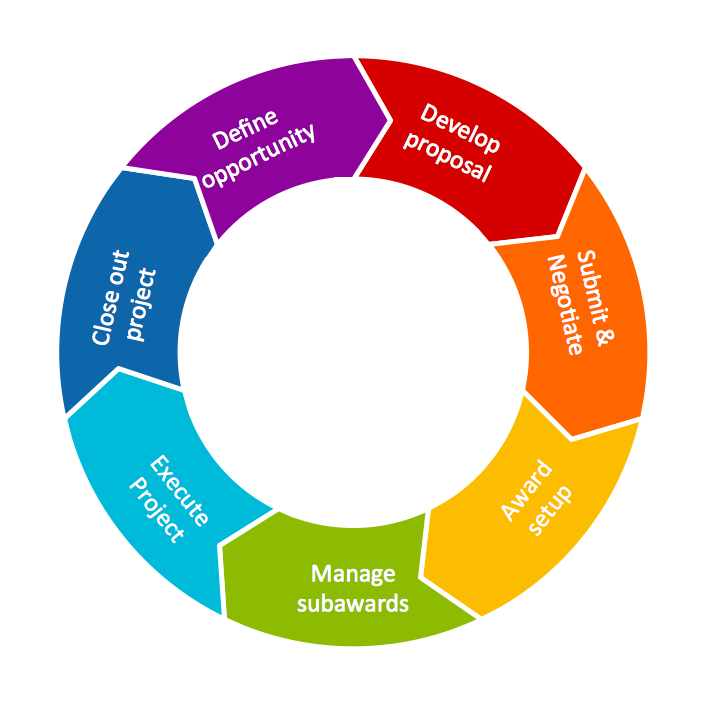Picture: Circular Diagram
Related Solution: